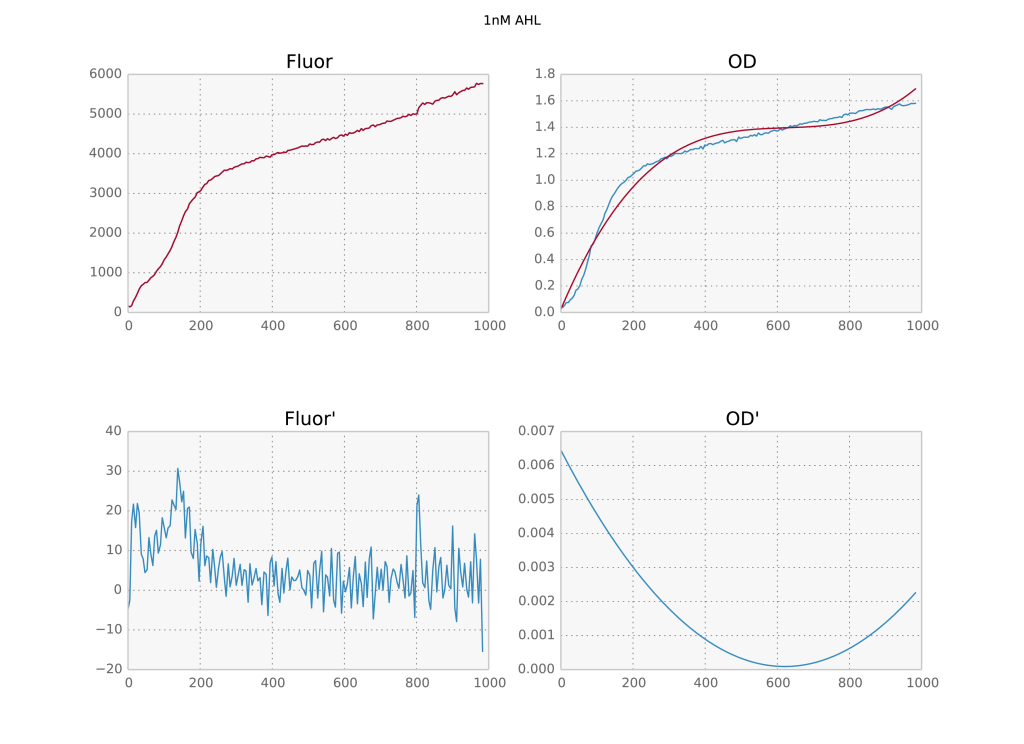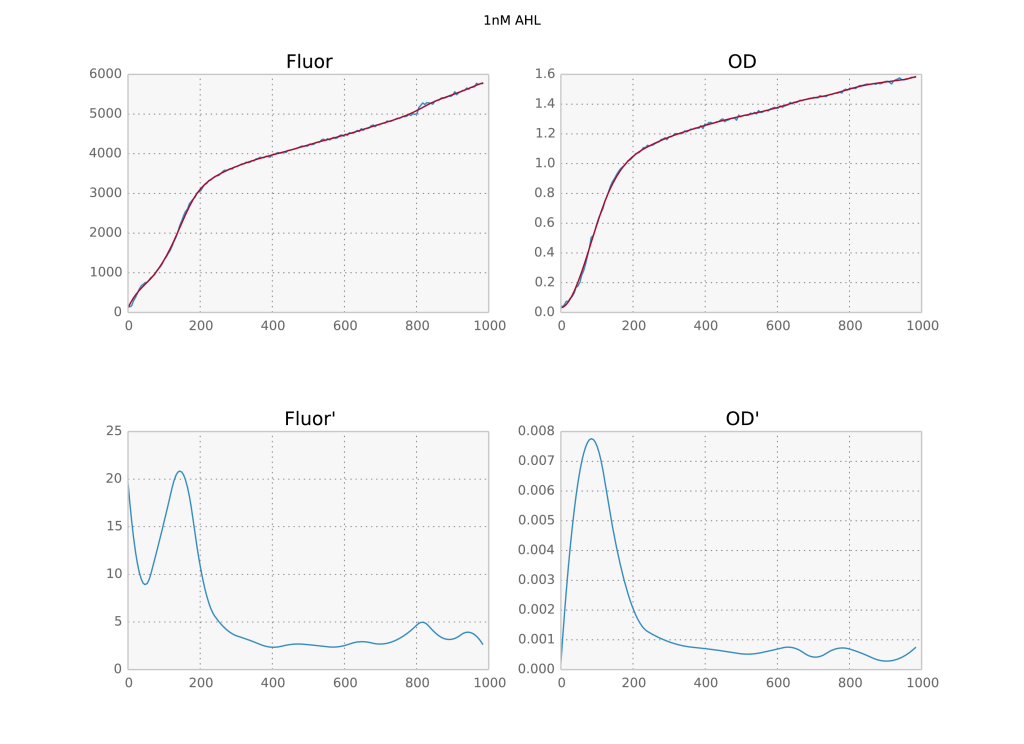# How to fix scipys interpolating spline default behavior

Scipy’s UnivariateSpline class is a super useful way to smooth time series, especially if you need an estimate of the derivative. It is an implementation of an interpolating spline, which I’ve previously covered in this blog post. Its big problem is that the default parameters suck. Depending on the absolute value of your data, the spline produced by leaving the parameters at their default values can be overfit, underfit or just fine. Below I visually reproduce the problem for two time series from an experiment with very different numerical values.Two time series with different numerical values and their derivatives below. The first is overfit, the second underfit.

My usual solution was just to manually adjust the $s$ parameter until the result looked good. But this time I have hundreds of time series, so I have to do it right this time. And doing it right requires actually understanding what’s going on. In the documentation, $s$ is described as follows:

Positive smoothing factor used to choose the number of knots. Number of knots will be increased until the smoothing condition is satisfied:

sum((w[i]*(y[i]-s(x[i])))**2,axis=0) <= s

If None (default), s=len(w) which should be a good value if 1/w[i] is an estimate of the standard deviation of y[i]. If 0, spline will interpolate through all data points.

So the default value of $s$ should be fine if $w^{-1}$ were an estimate of the standard deviation of $y$. However, the default value for $w$ is 1/len(y) which is clearly not a decent estimate. The solution then is to calculate a rough estimate of the standard deviation of $y$ and pass the inverse of that as $w$. My solution to that is to use a gaussian kernel to smooth the data and then calculate a smoothed variance as well. Code below:

def moving_average(self, series, sigma=3):
b = gaussian(39, sigma)
average = filters.convolve1d(series, b/b.sum())
var = filters.convolve1d(np.power(series-average,2), b/b.sum())
return average, var

_, var = moving_average(series)
sp = ip.UnivariateSpline(x, series, w=1/np.sqrt(var))Now, you may be thinking I only moved the parameter dependence around: before I had to fine tune $s$ but now there is a free parameter sigma. The difference is that a) the gaussian filter results are much more robust with respect to the choice of sigma;  b) we only need to provide an estimate of the standard deviation, so it’s fine if the result coming out is not perfect; and c) it does not depend on the absolute value of the data. In fact, for the above dataset I left sigma at its default value of 3 for all timeseries and all of them came out perfect. So I’d consider this problem solved.

I understand why the scipy developers wouldn’t use a method similar to mine to estimate $w$ as default, after all it may not work for all types of data. On the other hand, I think the documentation as it stands is confusing. The user would expect that parameters which have a default value would work without fine tuning, instead what happens here is that if you leave $w$ as the default you must change $s$ and vice versa.